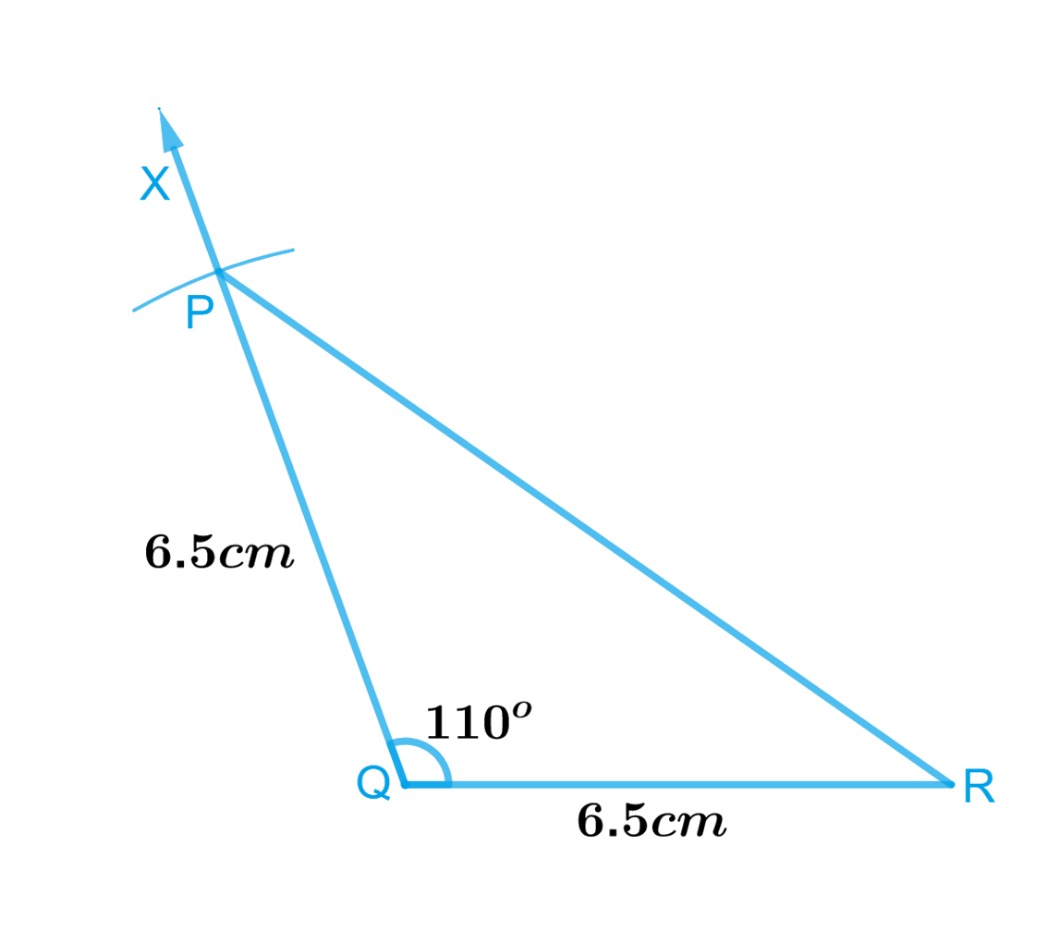# Ex.10.3 Q2 Practical Geometry Solution- NCERT Maths Class 7

Go back to  'Ex.10.3'

## Question

Construct an isosceles triangle in which the lengths of each of its equal sides is $$6.5\,\rm{ cm}$$ and the angle between them is $$110^\circ$$

Video Solution
Practical Geometry
Ex 10.3 | Question 2

## Text Solution

What is known?

Lengths of each of its equal sides is $$6.5\,\rm{ cm}$$ and the angle between them is $$110^\circ.$$

To construct:

An isosceles triangle in which the lengths of each of its equal sides is $$6.5\,\rm{ cm}$$ and the angle between them is $$110^\circ.$$

Reasoning:

To construct an isosceles triangle in which the lengths of each of its equal sides is $$6.5\,\rm{ cm}$$ and the angle between them is $$110^\circ,$$  follow the steps given below.

Steps:Steps of construction :

1. Draw a line segment $$QR$$ of length $$6.5\,\rm{cm}.$$
2. At $$Q,$$ draw $$QX$$ making an angle of $$110^\circ$$ with $$QR.$$
3. With $$Q$$ as centre, draw an arc of radius $$6.5\,\rm{cm}.$$ It cuts $$QX$$ at the point $$P.$$
4. Join $$PR$$. Triangle $$PQR$$ is the required isosceles triangle.

Learn from the best math teachers and top your exams

• Live one on one classroom and doubt clearing
• Practice worksheets in and after class for conceptual clarity
• Personalized curriculum to keep up with school